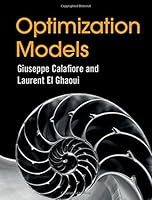# Optimization Models## Book Description

Emphasizing practical understanding over the technicalities of specific algorithms, this elegant textbook is an accessible introduction to the field of optimization, focusing on powerful and reliable convex optimization techniques. Students and practitioners will learn how to recognize, simplify, model and solve optimization problems - and apply these to their own projects. A clear and self-contained introduction to linear demonstrates core mathematical concepts in a way that is easy to follow, and helps students to understand their practical relevance. Requiring only a basic understanding of geometry, calculus, probability and statistics, and striking a careful balance between and rigor, it enables students to quickly understand the material, without being overwhelmed by complex . Accompanied by numerous end-of-chapter problems, an online solutions manual for instructors, and relevant examples from diverse fields including engineering, data science, economics, finance, and , this is the perfect introduction to optimization for undergraduate and graduate students.

Chapter 1 Introduction

Part I Models
Chapter 2 Vectors And Functions
Chapter 3 Matrices
Chapter 4 Symmetric Matrices
Chapter 5 Singular Value Decomposition
Chapter 6 Linear Equations And Least Squares
Chapter 7 Matrix Algorithms

Part II Convex Optimization Models
Chapter 8 Convexity
Chapter 9 Linear, Quadratic, And Geometric Models
Chapter 10 Second-Order Cone And Robust Models
Chapter 11 Semidefinite Models
Chapter 12 Introduction To Algorithms

Part III Applications
Chapter 13 Learning From Data
Chapter 14 Computational Finance
Chapter 15 Control Problems
Chapter 16 Engineering Design